[BACK]Structural Durability & Health MonitoringDOI: 10.32604/sdhm.2022.016905

ARTICLE

Aluminum Alloy Fatigue Crack Damage Prediction Based on Lamb Wave-Systematic Resampling Particle Filter Method

1College of Control Science and Engineering, Shandong University, Jinan, 250061, China
2Shandong Institute of Space Electronic Technology, Yantai, 264010, China
*Corresponding Author: Qingmei Sui. Email: qmsui@sdu.edu.cn
Received: 08 April 2021; Accepted: 21 July 2021

Abstract: Fatigue crack prediction is a critical aspect of prognostics and health management research. The particle filter algorithm based on Lamb wave is a potential tool to solve the nonlinear and non-Gaussian problems on fatigue growth, and it is widely used to predict the state of fatigue crack. This paper proposes a method of lamb wave-based early fatigue microcrack prediction with the aid of particle filters. With this method, which the changes in signal characteristics under different fatigue crack lengths are analyzed, and the state- and observation-equations of crack extension are established. Furthermore, an experiment is conducted to verify the feasibility of the proposed method. The Root Mean Square Error (RMSE) of the three different resampling methods are compared. The results show the system resampling method has the highest prediction accuracy. Furthermore, the factors affected by the accuracy of the prediction are discussed.

Keywords: Structural health monitoring; fatigue crack prognostics; particle filter; lamb wave; paris law

1  Introduction

Prognostics and health management (PHM) has recently become a novel engineering research hotspot , and it deals with the real-time assessment of a system under its practical operating conditions. Various surface-mounted sensors may be used in order to excite and collect a guided wave signal . When a guided wave signal acts on damage that exits inside the structure during propagation, it produces reflection and scattering. Then, according to the propagation characteristics of guided waves inside the structure, appropriate feature extraction technology can be used to analyze the collected wave signals that indicate damage information . Compared to traditional detection methods that may be unable to predict damage, PHM combines modern detection technology, modern digital signal processing technology, and machine learning , which greatly reduces the maintenance cost and time of the system [12,13].

Fatigue crack is an important aspect of PHM research, which significantly involves developing safety features as well as the extension of working time . Xu et al.  developed a novel method for fatigue crack identification based on nonlinear pseudo-force that appears at the location of a fatigue crack and vanished elsewhere. In their study, the ultrasonic nonlinear relative coefficient was used to quantitatively describe fatigue crack, which demonstrated that the nonlinear coefficient initially increased, after which it decreased with the increase of the fatigue crack . Lu et al.  quantitatively studied nonlinear parameter variation during crack growth via finite element simulation and experiment with a comparison of the results.

Due to complex work environments, however, the growth of fatigue cracks may be easily affected by a plethora of uncertain factors, such as material properties, temperature, unsteady force, and humidity [17,18]. Dodson et al.  developed the thermal sensitivity of dispersion curves and validated it. Abbas et al.  evaluated the impact of temperature on damage detection and provided an optimal baseline for thermal during ultrasonic lamb wave monitoring. Moreover, in most actual conditions, fatigue crack growth is nonlinear and exhibits non-Gaussian uncertainty. These uncertainties, in turn, increase the difficulty of fatigue crack monitoring. Hence, in order to deal with this level of uncertainty, a particle filter (PF) was introduced , which used a nonlinear state-space model and PF algorithm to evaluate the probability density function of the state, which demonstrated that the PF method was viable. Due to the combination of PF prediction methods with the crack growth law particle filter algorithm and online real-time monitoring, they do not need much test data, thus widening its scope for use in engineering.

With the development of non-destructive monitoring technology, PF, in conjunction with ultrasonic lamb wave detection, may be more suitable for application in engineering. Yang et al.  proposed a method of crack prognosis and compared it to Extended Kalman Filtering (EKF), which illustrated the superiority of the PF-based approach. Additionally, Chen et al.  used hole-edge crack specimens as a fatigue experiment object. According to the piezoelectric transducers (PZTs) active lamb, a method of online fatigue crack prognosis was presented, which adopted PF to deal with crack growth and monitor uncertainties. Neerukatti et al.  derived a methodology that combined a physics prediction model and data-driven localization method in order to estimate crack growth.

The aforementioned studies were based on the standard PF. Following particle propagation, weight computation, resampling, and the sample impoverishment  may be introduced, thereby increasing the number of particles that can deal with sample impoverishment . However, this solution costs requires additional time and more advanced hardware. Hol et al.  compared four kinds of resampling methods and analyzed the pros and cons of the four resampling algorithms according to the perspectives of resampling quality, computational complexity, and uniform distribution. Crack growth is a complicated process; predictive models are often established using an approximate method , and prediction models inevitably produce errors. The choice of resampling method is an important step in improving prediction accuracy.

Accordingly, this paper proposes an early fatigue microcrack damage prediction method based on the particle filter algorithm. Combined with finite element simulation, the variation in characteristic parameters when the wave passes through different crack lengths is then analyzed, while the damage index (DI) is used to observe crack length. The RMS of three different resampling methods is subsequently compared. Finally, the errors caused by the crack shape are discussed.

2  Framework of the Particle Filter Based on Fatigue Crack Growth

2.1 State-Space Model for Crack Growth

The state-space model based on the crack propagation law consists of a state equation and observation equation , as shown in Eq. (1).

{xk=f(xk1,wk1)yk=g(xk,vk) (1)

where xk−1 is the crack length at time k, f( ⋅ ) is defined by a crack growth model, through which the crack growth law from time k − 1 to k is started, wk−1 is the uncertainty of crack growth. yk is the observation value, which includes the fatigue crack information at the time k. g( ⋅ ) is a model, which is the relationship between the observation value and the crack length. vk refers to uncertainties during testing.

Paris’ law  is often used to describe the growth of fatigue crack, for which crack propagation is shown in Eq. (2).

dxdN=C(ΔK)m (2)

ΔK=Δσπx (3)

where N is the number of loading cycles, C and m are material parameters and ΔK is the stress intensity factor. The method  is simple to calculate. Eq. (2) is rewritten as

ln(dxdN)=ln(C)+mln(ΔK) (4)

The specimens are labeled as {Lj, j = 1, …, S}, the M group of crack measurement is collected {xij,i=1,,M} and the corresponding load cycles are labelled as {Nij,i=1,,M} , where x0j is the initial crack length. The crack growth rate can then be expressed as

(dxdN)ijΔxijΔNij=xijxi1jNijNi1j (5)

Given the crack growth rate {(dx/dN)ij,i=1,,M} and stress change factor ΔK, ln(Cj) and mj, which corresponds to the specimen Lj can be obtained through linear fitting.

The uncertainty estimate of crack propagation can be written as

ln(ΔxΔN)=ln[C(ΔK)m]+w (6)

eij=ln(ΔxijΔNij)ln{C[ΔK(xij)]m} (7)

where difference eij is assumed to be the uncertainty of crack growth, which affects the crack growth rate. Then, the difference between the samples can be used to calculate the variance σw2 of the random variable w.

σw2Var{eij,i=1,,M,j=1,,S} (8)

In order to consider uncertainty during the growth of fatigue crack as well as for simplification , Eq. (2) can be discretized as the state Eq. (9).

xk=xk1+C(ΔK)mΔN+w (9)

where ΔN is the discrete load step and w is the uncertainty of the growth crack, which follows N(0,σw2) .

Among the acquired monitored signals, the increase in the crack can cause signal distortion, energy attenuation, and phase delay . Accordingly, such characteristic parameters can be used to describe the current state of fatigue cracks. In order to quantify these features, the damage index (DI) was used, which evaluates the difference between the undamaged signal and the signal with fatigue load information. The results demonstrated that the S0 mode is more sensitive to early fatigue cracks compared to that of A0 mode . Therefore, the first wave packet (S0 mode) was used to calculate DI, which is given to Eq. (4).

DI=1CXYσXσY (10)

where CXY is the covariance of the two signals, σX and σY are the mean square deviations of the two signals, X is the baseline signal and Y is the monitored signal during crack propagation.

The corresponding observation is descriptive of the fatigue crack monitoring result, which can be obtained to fit all of the specimen’s DIs under different crack lengths, as shown in Eq. (11).

yk=b0+b1xk+b2xk2+b3xk3+vk (11)

where {b0, b1, b2, b3} are the coefficients of polynomial fitting.

The uncertainty of measurement is assumed to be normally distributed and is expressed as vN(0,σv2) . The damage factor variance of crack length xi can be expressed as

(σv2)iVar{yij,j=1,,S} (12)

2.2 Based on Different Resampling PF Methods

The standard PF was provided by the findings put forward by Gordon et al. , which was applied to the nonlinear and non-Gaussian problem. The PF was designed to calculate the posterior pdf p(xk | y1:k) with the specimen’s observations . The Bayes theorem is used to calculate the posterior pdf p(xk | y1:k), as expressed in Eq. (13).

{ p(xk1|y1:k1)=p(xk|xk1)p(xk1|y1:k1)dxk1p(xk|y1:k)=p(yk|xk)p(xk|y1:k1)p(yk|y1:k1) (13)

However, the pdf p(xk | y1:k) is actually difficult to calculate. The basic idea of the PF is to approximate the posterior pdf p(xk | y1:k) through a set of particles {xki,i=1,,N} with their normalized weights {w~ki,i=1,,N}

p(xk|y1:k)i=1Nw~kiδ(xkxki) (14)

where δ( ⋅ ) is the Dirac delta function, N is the number of particles, {xki,i=1,,N} are sampled from the pdf p(xk | y1:k).

When p(xk | y1:k) is unknown, the importance density function q(xk | y1:k) can then be introduced. Therefore, a posterior estimate can be expressed using Eq. (15), in which the particle weight is defined as in Eq. (16).

x^k=xkp(xk|y1:k)q(xk|y1:k)q(xk|y1:k)dxk (15)

wkip(xk|y1:k)q(xk|y1:k) (16)

The importance density function can be derived as

q(xk|y1:k)=q(xk1|yk)q(xk|xk1,y1:k) (17)

The unnormalized weight can be calculated through the iteration of Eq. (18).

wki=wk1ip(yk|xki)p(xki|xk1,y1:k)q(xki|y1:k) (18)

The weight normalization is shown in Eq. (19).

w~ki=wkii=1Nswki (19)

where p(yk|xki) is the likelihood value corresponding to the particle. Due to the simplicity of the conversion probability density function (PDF), the particle filter algorithm adopts the conversion probability density function as the importance density. Therefore, the weight update can be simplified into Eq. (20).

wki=wk1ip(yk|xki) (20)

The prognostic result  of the crack length xk is calculated as in Eq. (21).

x^k=i=1Nxkiw~ki (21)

The following describes three resampling methods:

(1)    Residual resampling 

ni=[Nwi] copies of the particle xi are allocated to the new distribution. Additionally, m=Nni particles are resampled from {xi} by making ni copies of particle xi where the probability for selecting xi is proportional to wi=Nwini using one of the aforementioned resampling schemes.

(2)    Systematic resampling 

N ordered numbers are then generated, as shown in Eq. (22).

uk=(k1)+u~N (22)

where u~U[0,1) , k = 1, 2, 3…N, which are then used to select xk according to the multinomial distribution.

Here,

xk=x(F1(uk)) (23)

where uk[s=1i1ws,s=1iws) , F−1 denotes the generalized inverse of the cumulative probability distribution of the normalized particle weights.

(3)    Multinomial resampling 

N ordered numbers are generated.

uk=uk+1u~k1k (24)

uN=u~N1N (25)

Here, u~kU[0,1) , which are used to select xk according to the multinomial distribution.

The resampling method involves eliminating particles that have small normalized importance weights and copying upon particles with large weights, setting all the weights to 1/N.

2.3 Framework of the Prediction Process

Fig. 1 shows the framework of the prediction process, which is roughly divided into three steps.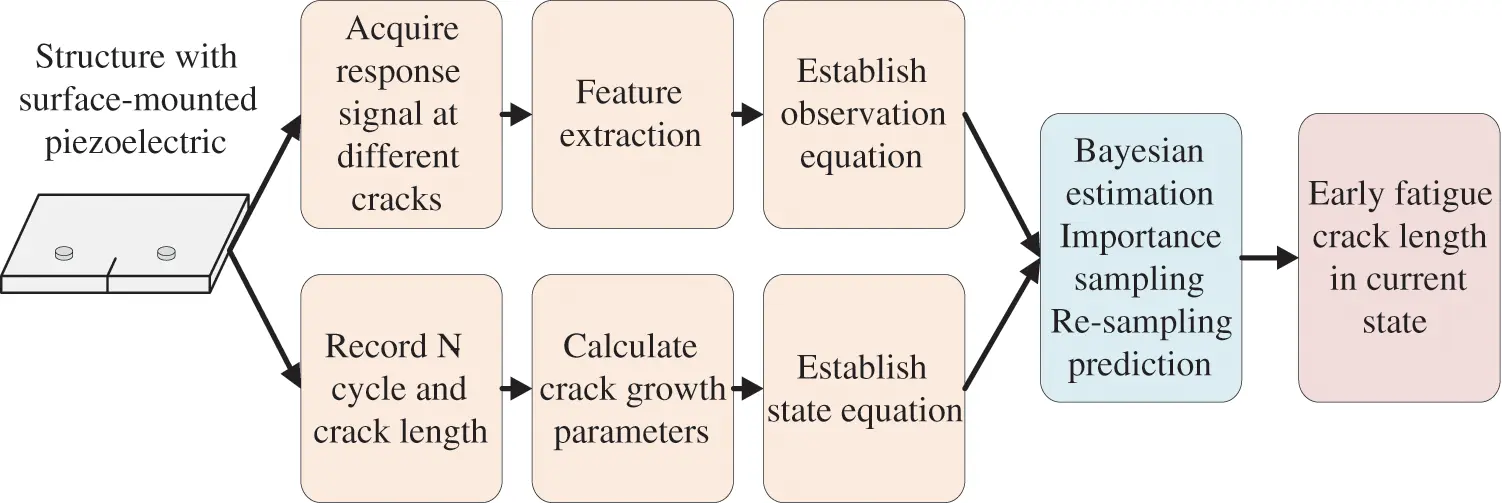Figure 1: Framework of the prediction process

Step 1. Record the number of loads and crack length during the fatigue crack growth process, calculate the crack growth parameters, and establish the crack growth state equation.

Step 2. Obtain response signals under different fatigue crack conditions via ultrasonic lamb wave detection technology, extract characteristic values, and establish observation equations.

Step 3. Use particle filter algorithm to realize early fatigue microcrack prediction.

3  Experimental Evaluation on Plates

3.1 Finite Element Simulation

In order to ascertain the energy change of lamb waves passing through different crack lengths, numerical experiments are performed using the finite element method (FEM). An aluminum plate with the encastre boundary condition is modeled using the ABAQUS software, as shown in Fig. 2.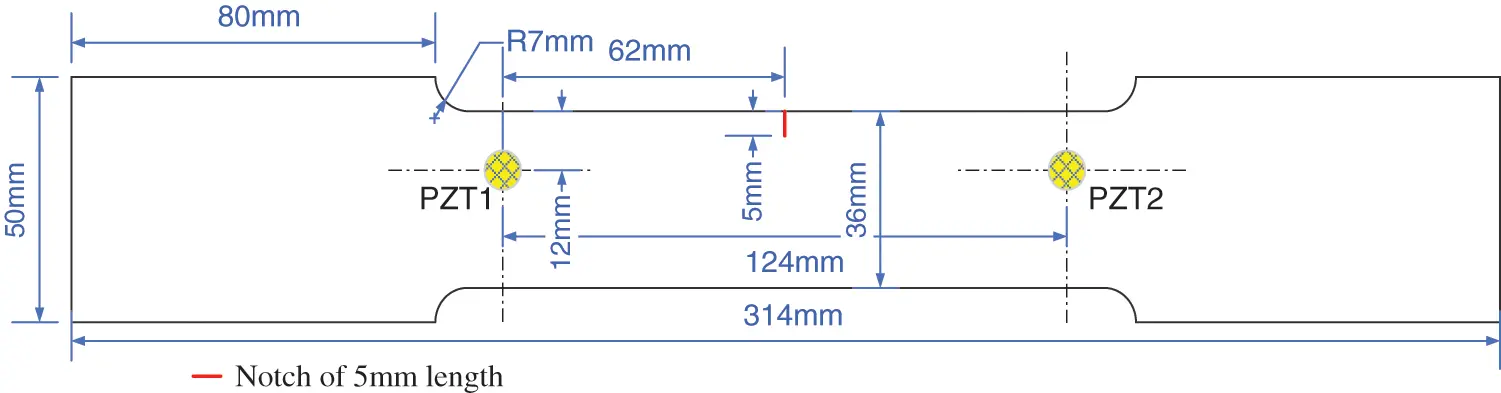Figure 2: Geometries of the specimen

A through-thickness crack with a width of 0.5 mm was modeled onto the side of the plate. In order to simulate early fatigue cracks, at the center of this crack, another size crack was cut out with a width of 0.1 mm and length varying from 0 to 12 mm, which had an increment of 3 mm, as shown in Fig. 3. The pitch-catch configuration was often used for damage detection, and the actuator and receiver were set on both sides of the damaged region.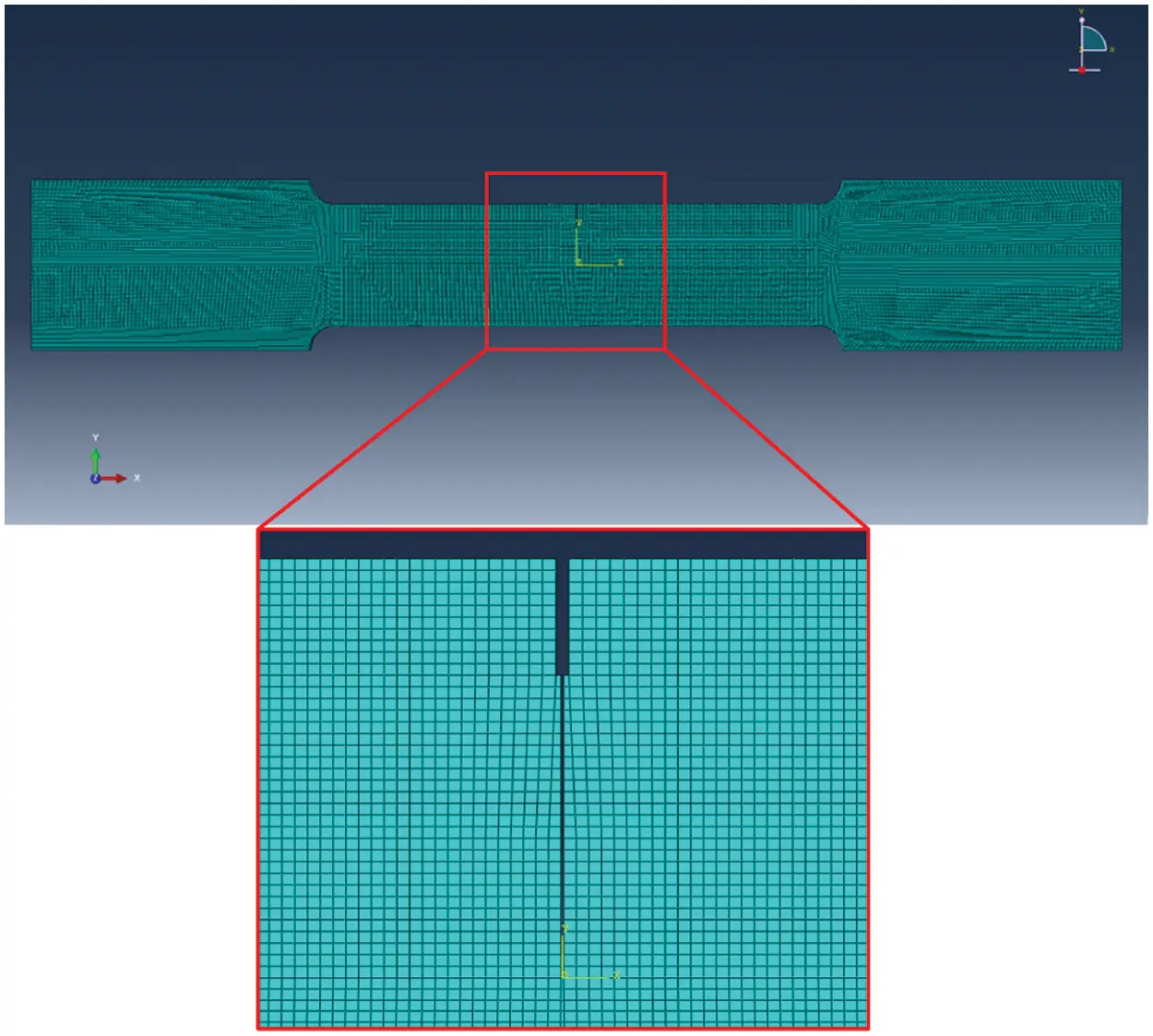Figure 3: Crack simulation diagram

According to the dispersion curve of Lamb wave propagating in a 6061-T6 aluminum alloy plate with 5 mm thickness, when the frequency thickness product is less than 2 MHzmm , the excited lamb wave possess only two modes, S0 and A0. The excitation frequency of the Lamb wave is then set as be 0.148 MHz, as shown in Fig. 4. The properties of the material are listed in Table 1.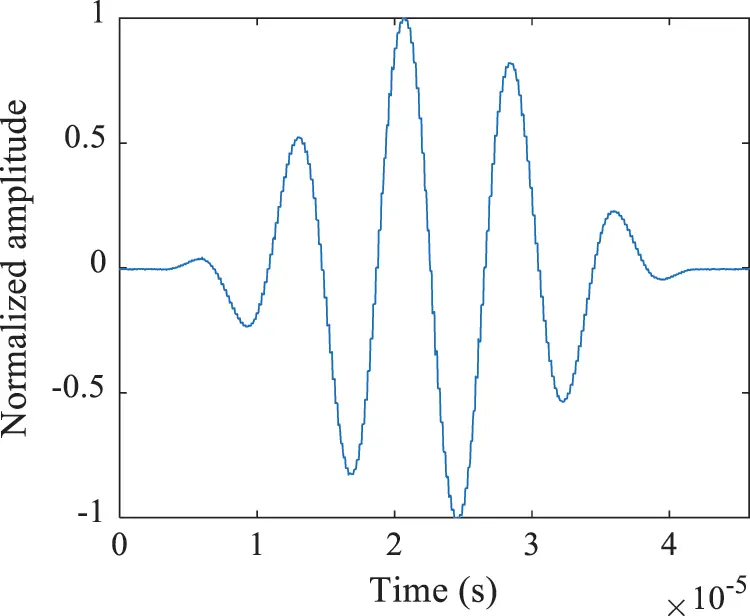Figure 4: 5-cycle excitation signal for lamb wave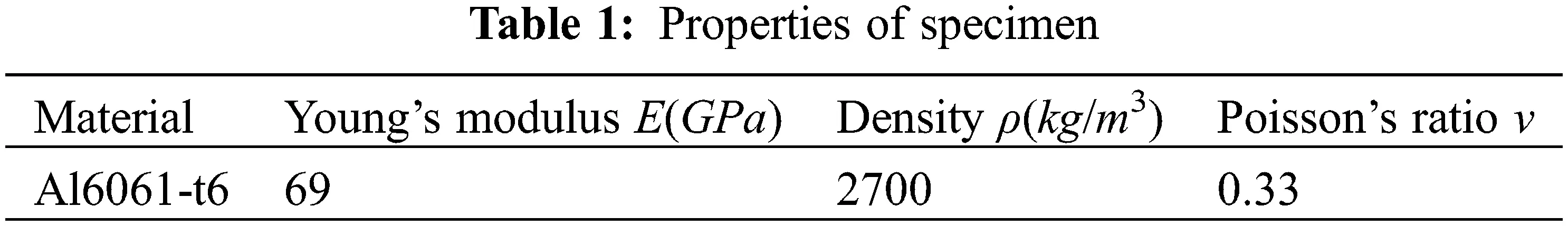Fig. 5 illustrates the response signal under different crack lengths. Fig. 6 shows that when the lamb wave is reflected when passing through the crack, the energy is attenuated, flight time becomes longer, and the whole waveform is distorted. In light of these three characteristics, fatigue cracks of different lengths can be designated.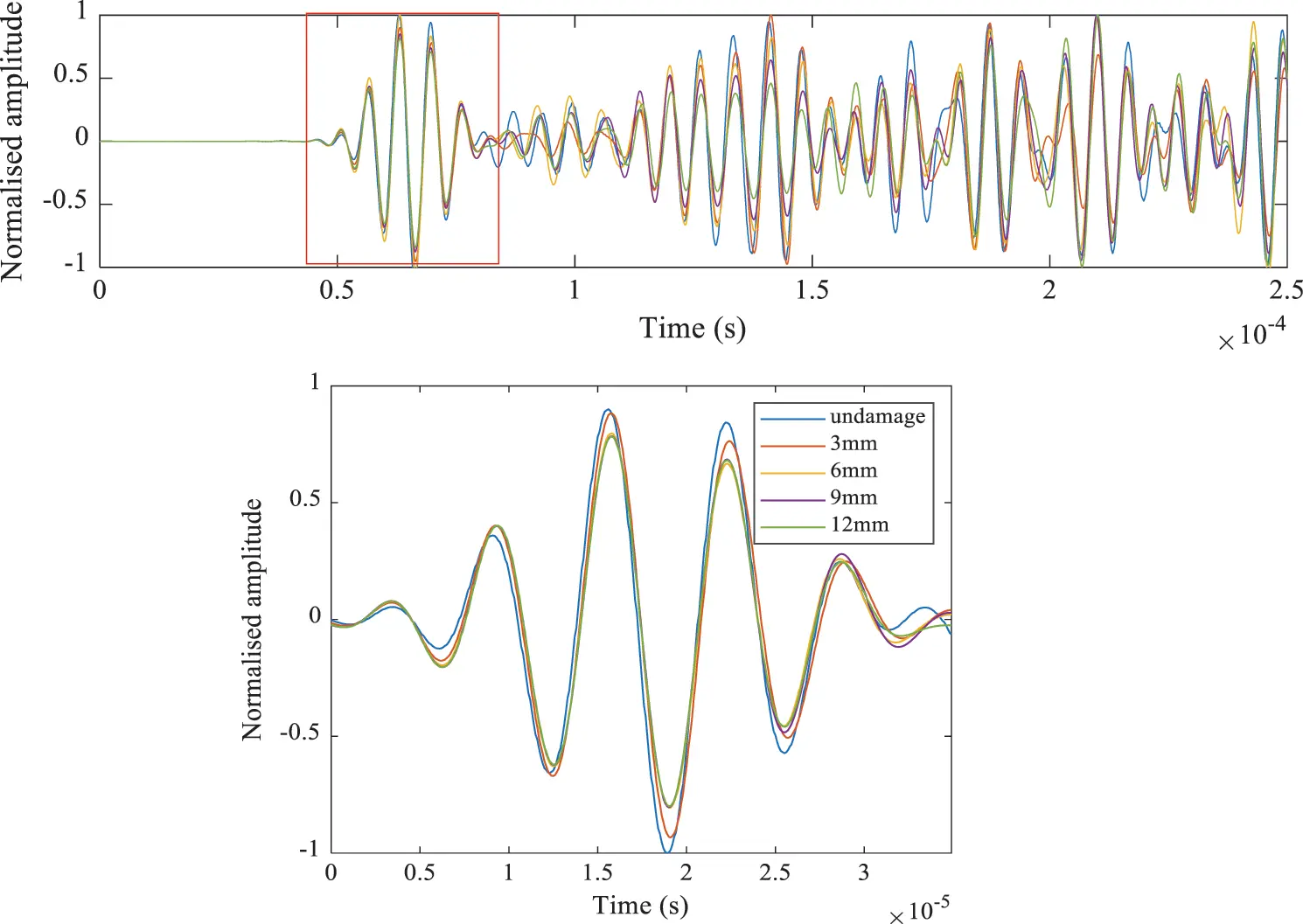Figure 5: FEM simulation response signals of different crack lengths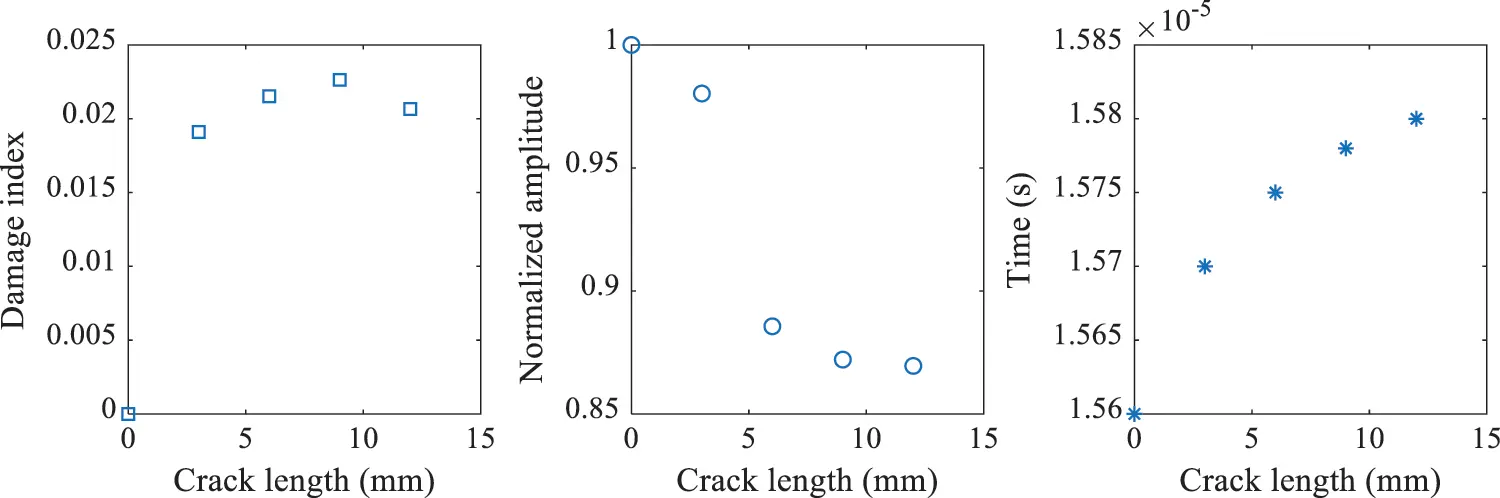Figure 6: Damage index, time, normalized amplitude under different length crack

3.2 Experimental Setup

With regard to verify the proposed method, a fatigue test for plate specimens consisting of 6061-t6 aluminum was performed. The thickness of all specimens was 5 mm, which were labeled from L1 to L5. A 5 mm notch was then machined at the specimen’s edge in order to initiate the direction of fatigue crack growth. As illustrated in Fig. 1, two sensors were adhered onto the plate specimen surface, and PZT1 was applied as the actuator to activate the lamb wave, while PZT2 was used as the sensor to acquire the wave signal. The crack specimens and sensor layouts are shown in Fig. 7.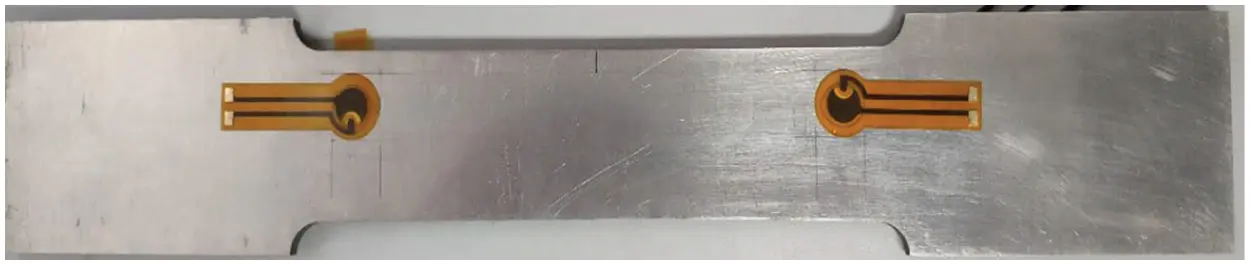Figure 7: Plate specimen

The Lamb wave based on the SHM technique was used for fatigue crack detection, which was sensitive to fatigue crack. The change in characteristics was due to discontinuities introduced by the growth of fatigue crack. These discontinuities can disperse and reflect the energy of the original Lamb wave, triggering changes in wave characteristics . The fatigue crack can then be identified by extracting feature changes from the Lamb wave signals.

A Multipurpose Servohydraulic Universal Testing Machine (Serises LFV 250 KN) was used to apply fatigue load. Specimen L1 was initially performed to determine the fatigue load with a fracture load of 35 KN. According to the results, a sinusoidal load with a peak value of 10 KN was chosen for specimens L2 to L5. The stress ratio was r = 0.1, and the frequency of fatigue load was selected as 15 Hz, as shown in Fig. 8a. Accordingly, a 5-cycle sine burst signal with 160 kHz central frequency and ±48 V amplitude was excited by PZT1. The Lamb wave signal was then acquired at a sampling rate of 256 MHz.Figure 8: (a) Fatigue tensile test setup, (b) The testing equipment for the fatigue specimens

During the fatigue test, a digital microscope was used to observe the growth of the crack. When the crack grew to about 1 mm, the specimen was taken down. The system was then used to monitor the fatigue specimen, as shown in Fig. 8b.

3.3 Fatigue Crack Growth and Test Results

Fig. 9 shows the L2 growth of the fatigue crack to 3 mm. The appearance of fatigue cracks caused discontinuities within the material structure as well as the energy attenuation and phase delay of Lamb waves passing through the discontinuous positions. Fig. 10 shows the waveform changes of the S0 mode under different fatigue crack lengths, while Fig. 11 shows the changes of the three typical characteristic parameters of DI, Normalized amplitude, and Time delay with the length of the fatigue cracks. The results demonstrated that the DI and phase increase with a rise in fatigue cracks, while the amplitude decreases with the increase of fatigue cracks. The results are noted to be consistent with the simulation analysis.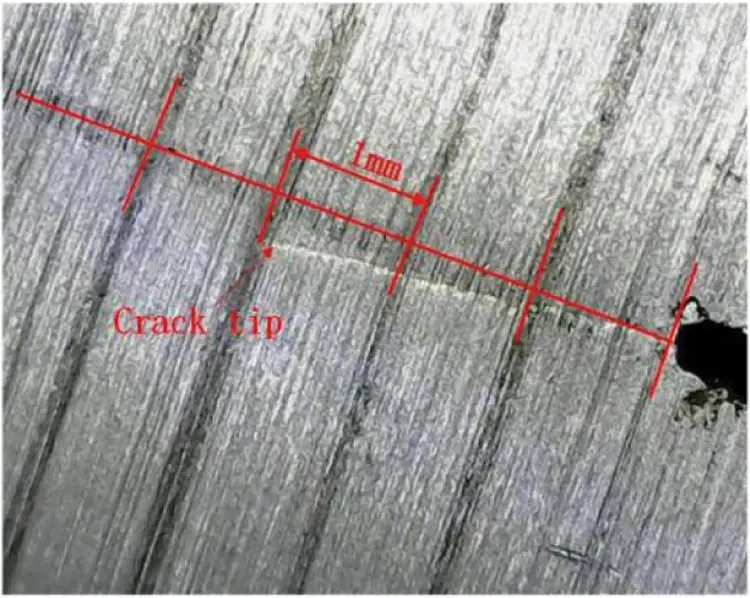Figure 9: The growth of fatigue crack to 3 mmFigure 10: Response signals of different crack lengths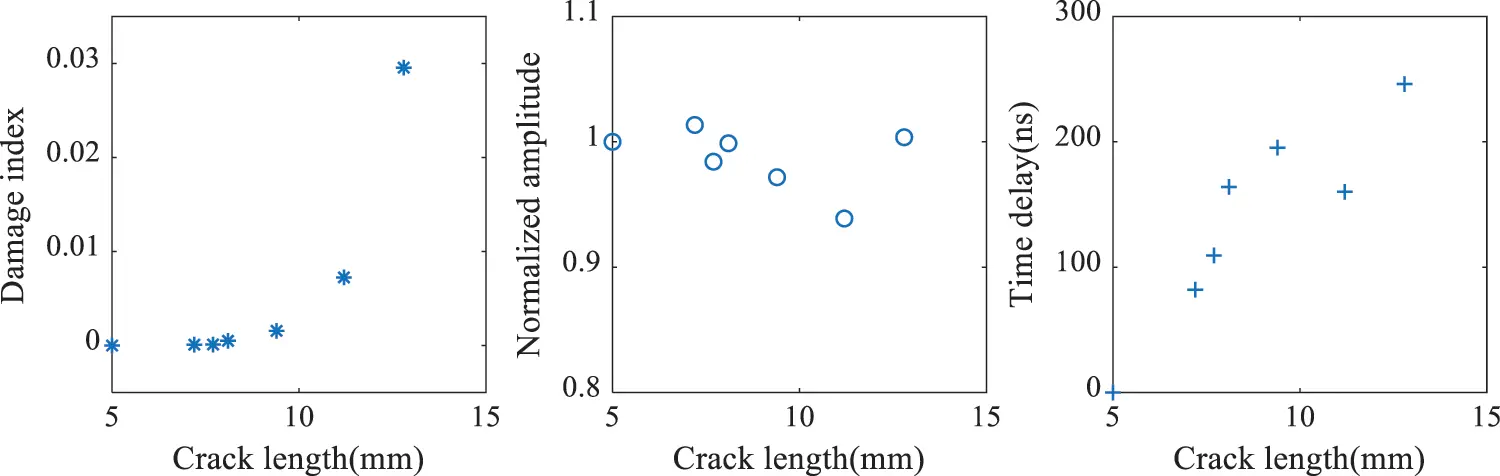Figure 11: Damage index, time delay, normalized amplitude under different length crack

Fig. 12a shows the crack growth curve of specimens L3–L5, in which the uncertainty of the material is evident. Fig. 12b describes the DIs under different fatigue crack conditions. Fig. 12c illustrates the DI curve of the specimens L3–L5 under different fatigue cracks, which was small during early fatigue crack growth. There were a number of uncertainties with the growth trajectories of the fatigue crack. According to the sensor arrangement, when the crack was small, the lamb wave of the S0 mode did not directly pass through the crack, having a lesser signal distortion.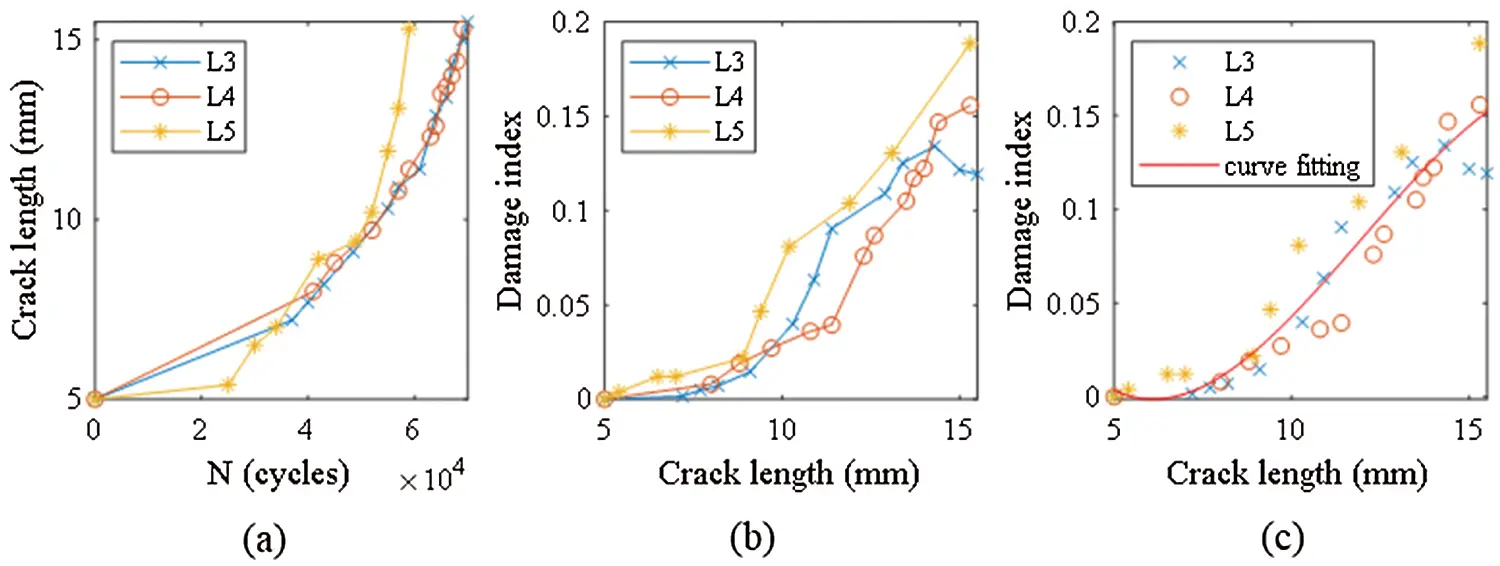Figure 12: (a) N-crack length curves. (b) Crack length-damage index. (c) Observation equation fitting curves

3.4 State-Space Model for the Plate

In order to verify the feasibility of the proposed method. L3–L5 specimens were used to establish the state-space model, while L3–L4 specimens underwent prognostic validation. As mentioned in Section 2.1, material parameters log C0, m and σw of specimens L3–L5 were calculated , as shown in Table 2.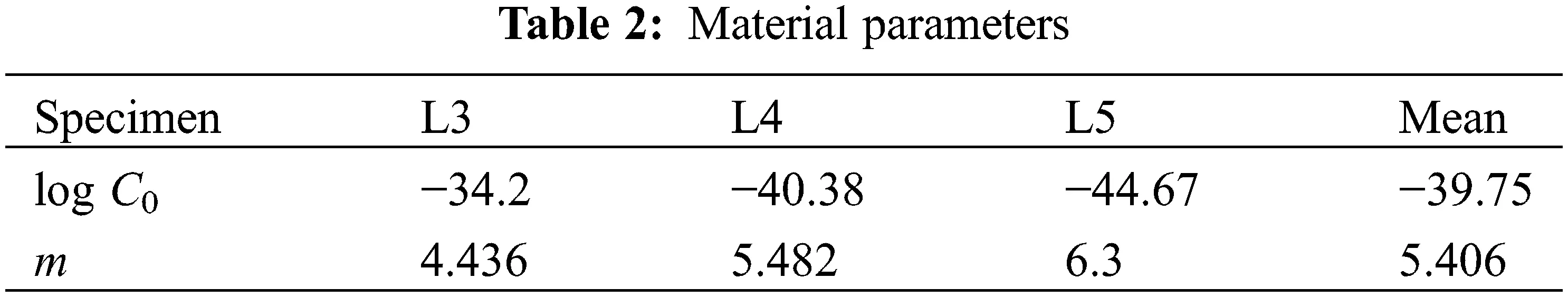The value of log C0 and m were obtained as log C0 = −39.75, m = 5.406, σw2=0.1692 , which were then applied to Eq. (9), where the discrete load step was set as ΔN = 50 and the particle number was set to 5000. According to Eq. (10), the DI data were calculated from the L3–L5 specimens. The curve fitting tool was then used to fit the observation equation, as shown in Fig. 12c. Furthermore, the RMSE of the fitting curve was selected as the observation uncertainty, which was 0.012 and v ∼ N(0, 0.012).

By substituting the calculation parameters into Eq. (1), the state and observation equation were obtained, as shown in Eq. (26).

{xk=xk1+C(ΔK)mΔN+wyk=0.000211xk3+0.0076xk20.0688xk+0.1846+v (26)

4  Result and Discussion

Figs. 13 and 14 show the predicted results of specimens L3, L4. Fig. 15 illustrates RMSE (Root Mean Square Error) under three resampling methods. According to the figure, the systematic resampling method possessed a small prediction error.Figure 13: The prognosis results of specimen L3. (a) Residual resampling. (b) Systematic resampling. (c) Multinomial resampling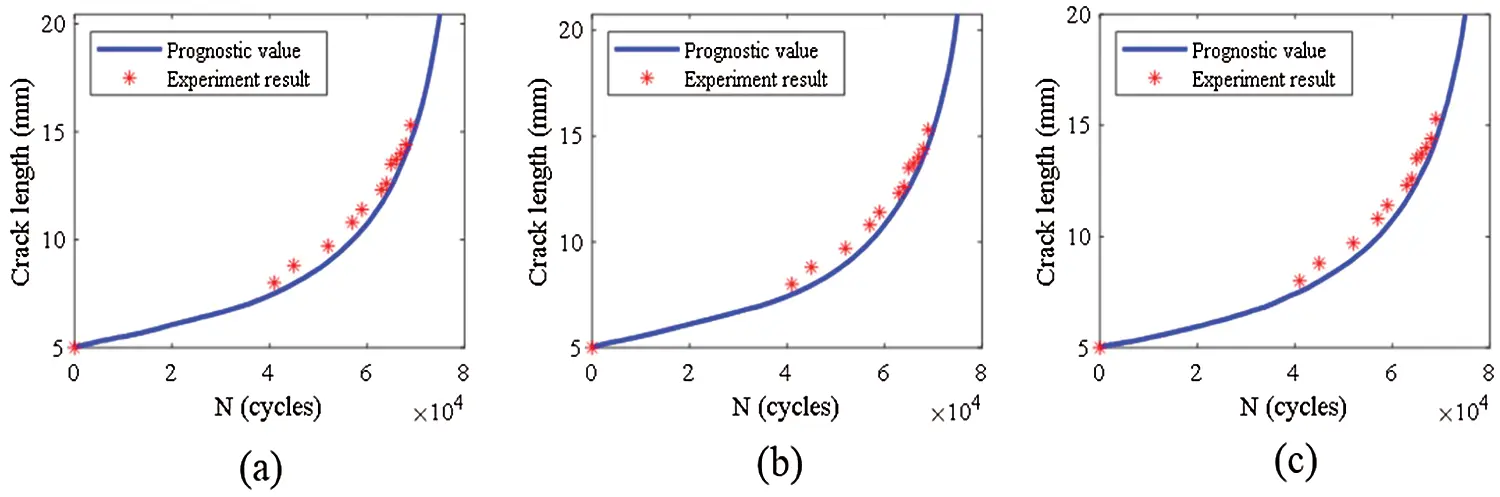Figure 14: The prognosis results of specimen L4. (a) Residual resampling. (b) Systematic resampling. (c) Multinomial resampling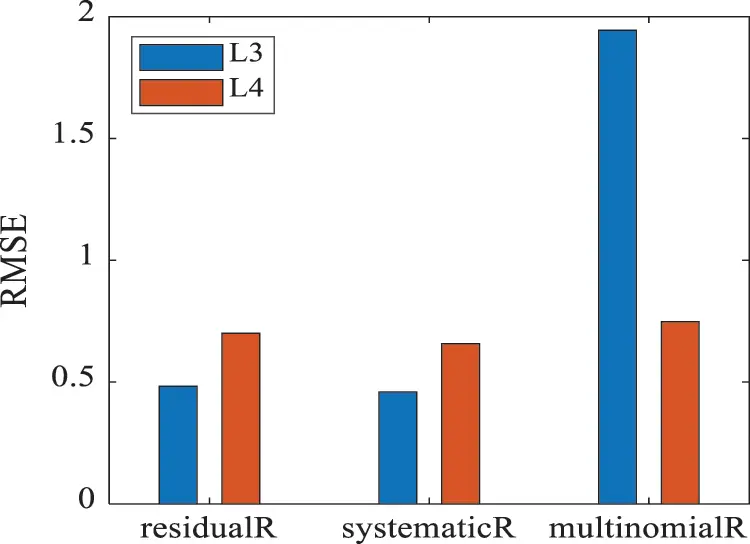Figure 15: The prediction RMS of specimen L3 and L4

DI was used to quantify the length of the crack, which was the energy attenuation and phase delay when the wave passed through the crack that was directed by the shape of the crack, affecting the accuracy of the observation. The fatigue crack is divided into three stages during growth: initiation, propagation, and fracture . The fatigue crack growth extended from a closed crack to an open crack, which then finally broke . Using Paris’ law to study crack growth and by calculating the material parameters, lgC and m were only considered in the Paris region (propagation). Moreover, initiation and fracture were not suitable for this law, increasing the error of fatigue crack prediction.

When the wave passed through a closed crack, wave A passed through directly, whereas wave B passed through the crack tip. The wave received by the piezoelectric plate was a superposition of these two waves. When the wave passes through an open crack, only wave B passed through the crack , as shown in Fig. 16. Regarding different specimens with the same crack length, when the wave passed through the fatigue crack, the energy attenuation and phase delay of waves A and B were different, resulting in different DIs. Moreover, the distance between the tip of the pre-crack as well as the propagation path had an effect on the DI of the fatigue crack . The closer the distance, the more sensitive the early fatigue crack DI changes with length. Meanwhile, the farther the distance, the less sensitive the early fatigue crack DI changes with length.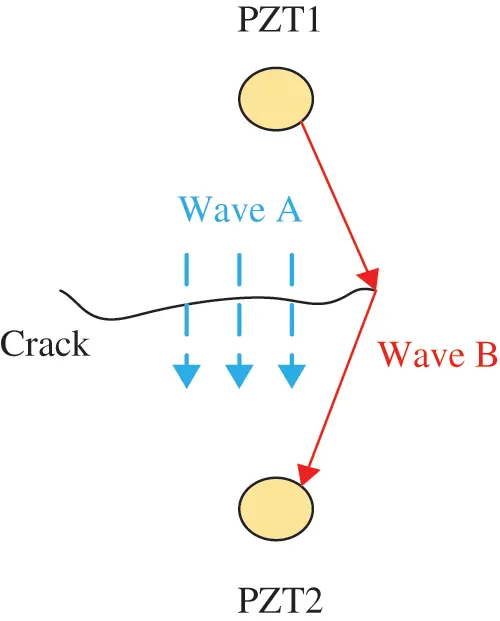Figure 16: Schematic diagram of wave propagation through the fatigue crack

5  Conclusions

This paper proposes an ultrasonic Lamb wave fatigue damage detection method according to the particle filter algorithm. Here, the change in eigenvalues when the lamb wave passes through the open crack is simulated by the finite element method. Additionally, this experiment analyzes the energy and phase changes of the S0 mode when passing through the crack. Accordingly, the findings of this study demonstrate that as the crack increases, the energy attenuation and DI rise, while the phase delay becomes longer. The simulation is found to be consistent with the results of the experiment. Moreover, the residual resample prediction errors of L3 and L4 are noted to be 0.48 and 0.7, while the systematic resample are 0.46 and 0.66, and the multinomial resamples are 1.94 and 0.75. The RMS under the three different resampling methods are then compared, in which systematic resampling is found to have a smaller prediction error. The results demonstrate that the proposed method is capable of effectively predicting fatigue crack propagation. Finally, the factors that may cause prediction errors are discussed. In view of the corresponding findings, conducting a further analysis on the propagation mode of Lamb waves under different crack types is needed. According to present results, the following conclusions were drawn:

1.    The increase of the growth fatigue crack has an obvious effect on the characteristic parameters of ultrasonic Lamb waves, which can be used to establish a fatigue crack observation model.

2.    The particle filter algorithm can be used well in order to solve the uncertainty in crack propagation and can realize the prediction of the fatigue crack.

3.    The prediction errors of the system resampling algorithm are 0.46 and 0.66, which are smaller than those of the other two resampling algorithms.

Funding Statement: This work was supported by the National Natural Science Foundation of China (62073193, 61903224, 61873333); National Key Research and Development Project (2018YFE02013); Key research and development plan of Shandong Province (2019TSLH0301, 2019GHZ004).

Conflicts of Interest: The authors declare that they have no conflicts of interest to report regarding the present study.

## References

1. Silik, A., Noori, M., Altabey, W. A., Ghiasi, R., & Wu, Z. (2021). Comparative analysis of wavelet transform for time-frequency analysis and transient localization in structural health monitoring. Structural Durability & Health Monitoring, 15(1), 1-22. [Google Scholar] [CrossRef]
2. Gao, D., Ma, Y., Wu, Z., Zheng, Y., & Lu, H. (2021). Guided wave based damage detection method for aircraft composite structures under varying temperatures. Structural Durability & Health Monitoring, 15(1), 23-37. [Google Scholar] [CrossRef]
3. Wandowski, T., Malinowski, P., & Ostachowicz, W. (2019). Elastic wave mode conversion phenomenon in glass fiber-reinforced polymers. International Journal of Structural Integrity, 10(3), 337-355. [Google Scholar] [CrossRef]
4. Chen, J., Yuan, S., Qiu, L., Wang, H., & Yang, W. (2018). On-line prognosis of fatigue crack propagation based on Gaussian weight-mixture proposal particle filter. Ultrasonics, 82, 134-144. [Google Scholar] [CrossRef]
5. Yang, Y., Ng, C. T., & Kotousov, A. (2019). Second-order harmonic generation of lamb wave in prestressed plates. Journal of Sound and Vibration, 460, 114903. [Google Scholar] [CrossRef]
6. Radecki, R., Su, Z., Cheng, L., Packo, P., & Staszewski, W. J. (2018). Modelling nonlinearity of guided ultrasonic waves in fatigued materials using a nonlinear local interaction simulation approach and a spring model. Ultrasonics, 84, 272-289. [Google Scholar] [CrossRef]
7. Wang, C. H., & Rose, L. R. F. (2003). Wave reflection and transmission in beams containing delamination and inhomogeneity. Journal of Sound and Vibration, 264(4), 851-872. [Google Scholar] [CrossRef]
8. Ihn, J. B., & Chang, F. K. (2004). Detection and monitoring of hidden fatigue crack growth using a built-in piezoelectric sensor/actuator network: I. Diagnostics. Smart Materials and Structures, 13(3), 609. [Google Scholar] [CrossRef]
9. An, D., Kim, N. H., & Choi, J. H. (2015). Statistical aspects in neural network for the purpose of prognostics. Journal of Mechanical Science and Technology, 29(4), 1369-1375. [Google Scholar] [CrossRef]
10. Sun, F., Wang, N., He, J., Guan, X., & Yang, J. (2017). Lamb wave damage quantification using GA-based LS-SVM. Materials, 10(6), 648. [Google Scholar] [CrossRef]
11. An, D., Kim, N. H., & Choi, J. H. (2015). Practical options for selecting data-driven or physics-based prognostics algorithms with reviews. Reliability Engineering & System Safety, 133, 223-236. [Google Scholar] [CrossRef]
12. Kim, N. H., An, D., Choi, J. H. (2016). Prognostics and health management of engineering systems. Switzerland: Springer International Publishing.
13. Gao, S., Dai, X., Hang, Y., Guo, Y., Ji, Q. (2018). Airborne wireless sensor networks for airplane monitoring system. Wireless Communications and Mobile Computing. DOI 10.1155/2018/6025825. [CrossRef]
14. Xu, W., Su, Z., Radzieński, M., Cao, M., & Ostachowicz, W. (2021). Nonlinear pseudo-force in a breathing crack to generate harmonics. Journal of Sound and Vibration, 492, 115734. [Google Scholar] [CrossRef]
15. Chen, H., Zhang, G., Fan, D., Fang, L., & Huang, L. (2020). Nonlinear lamb wave analysis for microdefect identification in mechanical structural health assessment. Measurement, 164, 108026. [Google Scholar] [CrossRef]
16. Lee, Y. F., Lu, Y., & Guan, R. (2020). Nonlinear guided waves for fatigue crack evaluation in steel joints with digital image correlation validation. Smart Materials and Structures, 29(3), 035031. [Google Scholar] [CrossRef]
17. Zhang, J., Liu, K., Luo, C., & Chattopadhyay, A. (2013). Crack initiation and fatigue life prediction on aluminum lug joints using statistical volume element–based multiscale modeling. Journal of Intelligent Material Systems and Structures, 24(17), 2097-2109. [Google Scholar] [CrossRef]
18. Zhang, J., & Johnston, J. (2014). Chattopadhyay A. Physics-based multiscale damage criterion for fatigue crack prediction in aluminium alloy. Fatigue & Fracture of Engineering Materials & Structures, 37(2), 119-131. [Google Scholar] [CrossRef]
19. Dodson, J. C., & Inman, D. J. (2013). Thermal sensitivity of lamb waves for structural health monitoring applications. Ultrasonics, 53(3), 677-685. [Google Scholar] [CrossRef]
20. Abbas, S., Li, F., Zhu, Y., & Tu, X. (2020). Experimental investigation of impact of environmental temperature and optimal baseline for thermal attenuation in structural health monitoring based on ultrasonic guided waves. Wave Motion, 93, 102474. [Google Scholar] [CrossRef]
21. Orchard, M. E., & Vachtsevanos, G. J. (2007). A particle filtering approach for on-line failure prognosis in a planetary carrier plate. International Journal of Fuzzy Logic and Intelligent Systems, 7(4), 221-227. [Google Scholar] [CrossRef]
22. Chen, J., Yuan, S., Qiu, L., Cai, J., & Yang, W. (2016). Research on a lamb wave and particle filter-based on-line crack propagation prognosis method. Sensors, 16(3), 320. [Google Scholar] [CrossRef]
23. Chen, J., Yuan, S., Wang, H., & Yang, W. (2019). On particle filter improvements for on-line crack growth prognosis with guided wave monitoring. Smart Materials and Structures, 28(3), 035011. [Google Scholar] [CrossRef]
24. Neerukatti, R. K., Hensberry, K., Kovvali, N., & Chattopadhyay, A. (2016). A novel probabilistic approach for damage localization and prognosis including temperature compensation. Journal of Intelligent Material Systems and Structures, 27(5), 592-607. [Google Scholar] [CrossRef]
25. Li, T., Bolic, M., & Djuric, P. M. (2015). Resampling methods for particle filtering: Classification, implementation, and strategies. IEEE Signal Processing Magazine, 32(3), 70-86. [Google Scholar] [CrossRef]
26. Doucet, A., Godsill, S., & Andrieu, C. (2000). On sequential monte carlo sampling methods for bayesian filtering. Statistics and Computing, 10(3), 197-208. [Google Scholar] [CrossRef]
27. Hol, J. D., Schon, T. B., Gustafsson, F. (2006). On resampling algorithms for particle filters. IEEE Nonlinear Statistical Signal Processing Workshop, pp. 79–82. DOI 10.1109/NSSPW.2006.4378824. [CrossRef]
28. Yang, W., & Gao, P. (2019). Lamb wave-minimum sampling variance particle filter-based fatigue crack prognosis. Sensors, 19(5), 1070. [Google Scholar] [CrossRef]
29. Paris, P., Erdogan, F. (1963). A critical analys. Journal of Fluids Engineering, 85 (4), 528–533. DOI 10.1115/1.3656900. [CrossRef]
30. Yang, W., Yuan, S., & Chen, J. (2017). Application of deterministic resampling particle filter to fatigue prognosis. Journal of Vibroengineering, 19(8), 5978-5991. [Google Scholar] [CrossRef]
31. Lu, Y., Ye, L., Su, Z., & Yang, C. (2008). Quantitative assessment of through-thickness crack size based on lamb wave scattering in aluminum plates. NDT & E International, 41(1), 59-68. [Google Scholar] [CrossRef]
32. Yang, Y., Ng, C. T., Kotousov, A., Sohn, H., & Lim, H. J. (2018). Second harmonic generation at fatigue cracks by low-frequency lamb waves: Experimental and numerical studies. Mechanical Systems and Signal Processing, 99, 760-773. [Google Scholar] [CrossRef]
33. Gordon, N. J., Salmond, D. J., & Smith, A. F. M. (1993). Novel approach to nonlinear/non-Gaussian Bayesian state estimation. IEE Proceedings F (Radar and Signal Processing), 140(2), 107-113. [Google Scholar] [CrossRef]
34. Yuan, S., Chen, J., Yang, W., & Qiu, L. (2017). On-line crack prognosis in attachment lug using lamb wave-deterministic resampling particle filter-based method. Smart Materials and Structures, 26(8), 085016. [Google Scholar] [CrossRef]
35. Douc, R., Cappé, O. (2005). Comparison of resampling schemes for particle filtering. Proceedings of the 4th International Symposium on Image and Signal Processing and Analysis, pp. 64–69. DOI 10.1109/ISPA.2005.195385. [CrossRef]
36. He, J., Guan, X., Peng, T., Liu, Y., & Saxena, A. (2013). A multi-feature integration method for fatigue crack detection and crack length estimation in riveted lap joints using lamb waves. Smart Materials and Structures, 22(10), 105007. [Google Scholar] [CrossRef]
37. Liu, W., Wu, D., Duan, S., Wang, T., & Zou, Y. (2020). A study on fatigue crack propagation for friction stir welded plate of 7N01 Al-Zn-Mg alloy by EBSD. Materials, 13(2), 330. [Google Scholar] [CrossRef]
38. Shen, Y., & Giurgiutiu, V. (2014). Predictive modeling of nonlinear wave propagation for structural health monitoring with piezoelectric wafer active sensors. Journal of Intelligent Material Systems and Structures, 25(4), 506-520. [Google Scholar] [CrossRef]This work is licensed under a Creative Commons Attribution 4.0 International License, which permits unrestricted use, distribution, and reproduction in any medium, provided the original work is properly cited.